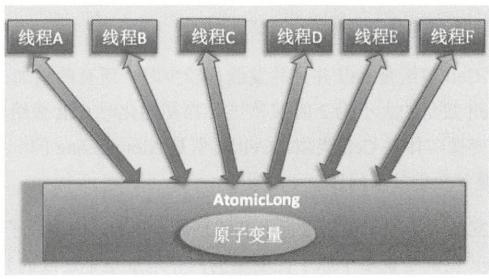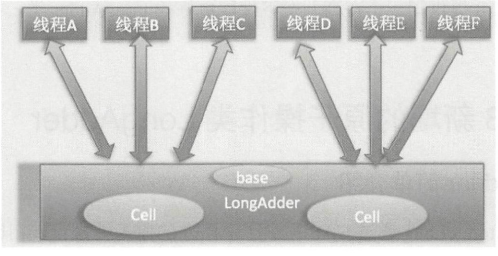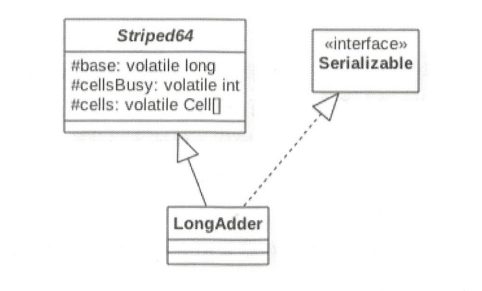• 累计撰写 147 篇文章
• 累计创建 43 个标签
• 累计收到 4 条评论

# Java并发编程（十七）Java并发包中原子操作类原理2021-02-21 / 0 评论 / 0 点赞 / 347 阅读 / 17,872 字 / 正在检测是否收录...

JUC包提供了一系列的原子性操作类，这些类都是使用非阻塞算法CAS 实现的，相比使用锁实现原子性操作这在性能上有很大提高。

# AtomicLong

AtomicLong是原子性递增或者递减类，其内部使用 Unsafe 来实现，前面的博文中有Unsafe类的介绍（Java 中的原子性操作），了解这个后再来看原子类源码就方便多了，下面我们直接上源码（AtomicLong）：

public class AtomicLong extends Number implements java.io.Serializable {
private static final long serialVersionUID = 1927816293512124184L;

// 获取 Unsafe 实例
private static final Unsafe unsafe = Unsafe.getUnsafe();
// value 变量在AtomicLong 类中的偏移量
private static final long valueOffset;

// 判断 JVM 是否支持 Long 类型无锁CAS
static final boolean VM_SUPPORTS_LONG_CAS = VMSupportsCS8();
private static native boolean VMSupportsCS8();

static {
try {
// 获取value 在 AtomicLong 中的偏移量
valueOffset = unsafe.objectFieldOffset
(AtomicLong.class.getDeclaredField("value"));
} catch (Exception ex) { throw new Error(ex); }
}
// 实际变量值，是声明为volatile哦，保证多线程下的内存可见性
private volatile long value;

public AtomicLong(long initialValue) {
value = initialValue;
}

....
}


## AtomicLong 中的主要方法

### 递增和递减操作代码

// 调用unsafe方法，原子性设置value值为原始值+1，返回值为原始值
public final long getAndIncrement() {
}
// 调用unsafe方法，原子性设置value值为原始值-1，返回值为原始值
public final long getAndDecrement() {
}
// 调用unsafe方法，原子性设置value值为原始值+1，返回值为递增之后的值
public final long incrementAndGet() {
return unsafe.getAndAddLong(this, valueOffset, 1L) + 1L;
}
// 调用unsafe方法，原子性设置value值为原始值-1，返回值为递增之后的值
public final long decrementAndGet() {
return unsafe.getAndAddLong(this, valueOffset, -1L) - 1L;
}


Unsafe.java

public final long getAndAddLong(Object obj,long offset,long addValue) {
long l;
do {
l = getLongvolatile (obj,offset);
return l;
}


### compareAndSet 方法

public final boolean compareAndSet(long expect, long update) {
return unsafe.compareAndSwapLong(this, valueOffset, expect, update);
}


public class AtomicLongTest {

/** 原子计数器 */
private static AtomicLong atomicLong = new AtomicLong();

/** 数据源 */
private static Integer[] arrayOne = new Integer[]{0, 1, 2, 3, 0, 6, 2, 8, 0, 4};
private static Integer[] arrayTwo = new Integer[]{7, 10, 0, 3, 6, 0, 2, 0, 0, 7};

public static void main(String[] args) throws InterruptedException {
// threadOne 统计 arrayOne 数组中 0 的个数
@Override
public void run() {
int size = arrayOne.length;
for (int i = 0; i < size; i++) {
if (arrayOne[i].intValue() == 0) {
atomicLong.incrementAndGet();
}
}
}
});

// threadTwo 统计 arrayTwo 数组中 0 的个数
@Override
public void run() {
int size = arrayTwo.length;
for (int i = 0; i < size; i++) {
if (arrayTwo[i].intValue() == 0) {
atomicLong.incrementAndGet();
}
}
}
});

System.out.println("count 0: " + atomicLong.get());
}
}

count 0: 7


AtomicLong 通过 CAS 提供了非阻塞的原子性操作，相比使用阻塞算法的同步器来说它的性能已经很好了，但是AtomicLong在高并发下大量线程会同时去竞争更新同一个原子变量，但是由于同时只有一个线程的 CAS操作会成功，这就造成了大量线程竞争失败后，会通过无限循环不断进行自旋尝试 CAS 的操作，而这会白白浪费 CPU 资源。

AtomicLong：多个线程同时竞争同一个原子变量2. 当前线程应该访问 Cell 数组里面的哪一个Cell元素？
3. 如何初始化 Cell 数组？
4. Cell数组如何扩容？
5. 线程访问分配的 Cell元素有冲突后如何处理？
6. 如何保证线程操作被分配的 Cell 元素的原子性？Striped64.java

// 1. LongAdder 的结构是怎样的？
// @sun.misc.Contended 注解，避免伪共享
@sun.misc.Contended static final class Cell {
// 内部维护了一个volatile 声明的value 变量
volatile long value;
Cell(long x) { value = x; }
// 6. 如何保证线程操作被分配的 Cell 元素的原子性？
// cas操作，保证线程更新Call中value变量的原子性
final boolean cas(long cmp, long val) {
return UNSAFE.compareAndSwapLong(this, valueOffset, cmp, val);
}

// Unsafe mechanics
private static final sun.misc.Unsafe UNSAFE;
private static final long valueOffset;
static {
try {
UNSAFE = sun.misc.Unsafe.getUnsafe();
Class<?> ak = Cell.class;
valueOffset = UNSAFE.objectFieldOffset
(ak.getDeclaredField("value"));
} catch (Exception e) {
throw new Error(e);
}
}
}


• long sum()
返回当前的值，内部操作是累加所有Cell内部的 value值后再累加 base。
由于计算总和时没有对 Cell 数组进行加锁，所以在累加过程中可能有其他线程对 Cell中的值进行了修改，也有可能对数组进行了扩容，所以sum返回的值并不是非常精确的，其返回值并不是一个调用 sum方法时的原子快照值。
public long sum() {
Cell[] as = cells; Cell a;
long sum = base;
if (as != null) {
for (int i = 0; i < as.length; ++i) {
if ((a = as[i]) != null)
sum += a.value;
}
}
return sum;
}

• long longValue()
调用sum()
• void reset()
为重置操作，如下代码把 base置为0，如果 Cell数组有元素，则元素值被重置为0。
public void reset() {
Cell[] as = cells; Cell a;
base = 0L;
if (as != null) {
for (int i = 0; i < as.length; ++i) {
if ((a = as[i]) != null)
a.value = 0L;
}
}
}

• long sumThenReset()
sum 的改造版本，如下代码在使用sum累加对应的Cell值后，把当前Cell的值重置为0，base 重置为0。这样，当多线程调用该方法时会有问题，比如考虑第一个调用线程清空 Cell的值，则后一个线程调用时累加的都是0值。
public long sumThenReset() {
Cell[] as = cells; Cell a;
long sum = base;
base = 0L;
if (as != null) {
for (int i = 0; i < as.length; ++i) {
if ((a = as[i]) != null) {
sum += a.value;
a.value = 0L;
}
}
}
return sum;
}

重要方法
public void add(long x) {
Cell[] as; long b, v; int m; Cell a;
// (1) 看cells是否为 null，如果为 null 则当前在基础变量 base上进行累加
if ((as = cells) != null || !casBase(b = base, b + x)) {
// 默认非竞争状态
boolean uncontended = true;
if (as == null || (m = as.length - 1) < 0 || // (2)
// 2. 当前线程应该访问 Cell 数组里面的哪一个Cell元素？
(a = as[getProbe() & m]) == null || // (3) 当前线程应该访问cells数组里面的哪一个Cell元素（getProbe() & m 确定了下标）
// 6. 如何保证线程操作被分配的 Cell 元素的原子性？
!(uncontended = a.cas(v = a.value, v + x))) // (4) 当前线程映射的元素存在, 使用 CAS操作去更新分配的 Cell元素的value值, 更新失败uncontended 标志为false 为竞争状态
// 如果当前线程映射的元素不存在或者存在但是CAS操作失败
longAccumulate(x, null, uncontended); // (5)
}
}

// Striped64类的方法
final boolean casBase(long cmp, long val) {
return UNSAFE.compareAndSwapLong(this, BASE, cmp, val);
}
// 用于获取当前线程中变量 threaLocalRandomProbe的值，这个值一开始为0, 在longAccumulate方法中会对其进行初始化
static final int getProbe() {
}
// 调整大小或创建单元时使用的自旋锁（通过CAS锁定）。
transient volatile int cellsBusy;
// cells 数组被初始化和扩容
final void longAccumulate(long x, LongBinaryOperator fn,
boolean wasUncontended) {
int h;
if ((h = getProbe()) == 0) {
h = getProbe();
wasUncontended = true;
}
boolean collide = false;                // 如果最后一个插槽非空则为真
for (;;) {
Cell[] as; Cell a; int n; long v;
if ((as = cells) != null && (n = as.length) > 0) { // (7)
if ((a = as[(n - 1) & h]) == null) { // (8)
if (cellsBusy == 0) {       // Try to attach new Cell
Cell r = new Cell(x);   // Optimistically create
if (cellsBusy == 0 && casCellsBusy()) {
boolean created = false;
try {               // Recheck under lock
Cell[] rs; int m, j;
if ((rs = cells) != null &&
(m = rs.length) > 0 &&
rs[j = (m - 1) & h] == null) {
rs[j] = r;
created = true;
}
} finally {
cellsBusy = 0;
}
if (created)
break;
continue;           // Slot is now non-empty
}
}
collide = false;
}
else if (!wasUncontended)       // CAS already known to fail
wasUncontended = true;      // Continue after rehash
// (9) 当前Cell存在 ，如 执行CAS设置
else if (a.cas(v = a.value, ((fn == null) ? v + x :
fn.applyAsLong(v, x))))
break;
// (10) 当前Cell数组元素个数大于CPU个数
else if (n >= NCPU || cells != as)
collide = false;            // At max size or stale
// (11) 是否有冲突
else if (!collide)
collide = true;
// (12) 如果当前元素个数没有达到 CPU 个数并且有冲突则扩容
// 这里的扩容操作也是先通过CAS 设置cellBusy为1，然后才能进行扩容。
else if (cellsBusy == 0 && casCellsBusy()) {
try {
if (cells == as) {      // Expand table unless stale
// 4. Cell数组如何扩容？
// (12.1)
Cell[] rs = new Cell[n << 1];
for (int i = 0; i < n; ++i)
rs[i] = as[i];
cells = rs;
}
} finally {
// (12.2)
cellsBusy = 0;
}
// (12.3)
collide = false;
continue;                   // Retry with expanded table
}
// (13） 为了能够找到一个空闲的Cell，重新计算hash值，xorshift算法生成随机数
}
// (14) 初始化Cell数组
else if (cellsBusy == 0 && cells == as && casCellsBusy()) {
boolean init = false;
try {                           // Initialize table
if (cells == as) {
// (14.1)
Cell[] rs = new Cell;
// (14.2)
rs[h & 1] = new Cell(x);
cells = rs;
init = true;
}
} finally {
// (14.3)
cellsBusy = 0;
}
if (init)
break;
}
else if (casBase(v = base, ((fn == null) ? v + x :
fn.applyAsLong(v, x))))
break;                          // Fall back on using base
}
}

/**
* 将cellBusy字段从0设为1，以获取锁定。
*/
final boolean casCellsBusy() {
return UNSAFE.compareAndSwapInt(this, CELLSBUSY, 0, 1);
}


cells数组的初始化是在代码 (14) 中进行的，其中cellsBusy是一个标示，为0说明当前cells数组没有在被初始化或者扩容，也没有在新建Cell元素，为1则说明cells数组在被初始化或者扩容，或者当前在创建新的 Cell元素、通过CAS操作来进行0或1状态的切换，这里使用 casCellsBusy 函数。假设当前线程通过CAS设置cellsBusy为1，则当前线程开始初始化操作，那么这时候其他线程就不能进行扩容了。如代码 (14.1) 初始化 cells数组元素个数为2，然后使用h&1计算当前线程应该访问cell数组的哪个位置，也就是使用当前线程的threadLocalRandomProbe变量值&(cells数组元素个数-1），然后标示cells 数组已经被初始化，最后代码 (14.3) 重置了cellBusy 标记。显然这里没有使用 CAS操作，却是线程安全的，原因是cellsBusyvolatile类型的，这保证了变量的内存可见性，另外此时其他地方的代码没有机会修改cellsBusy的值。在这里初始化的 cells数组里面的两个元素的值目前还是 null。这里回答了问题3，知道了cells数组如何被初始化。

cells数组的扩容是在代码 (12) 中进行的，对cells扩容是有条件的，也就是代码 (10) (11) 的条件都不满足的时候。具体就是当前cells的元素个数小于当前机器CPU个数并且当前多个线程访问了cells 中同一个元素，从而导致冲突使其中一个线程 CAS失败时才会进行扩容操作。这里为何要涉及CPU个数呢？

## 使用示例

public class LongAdderTest {

/**
* 数据源
*/
private static Integer[] arrayOne = new Integer[]{0, 1, 2, 3, 0, 6, 2, 8, 0, 4};
private static Integer[] arrayTwo = new Integer[]{7, 10, 0, 3, 6, 0, 2, 0, 0, 7};

public static void main(String[] args) throws InterruptedException {
// threadOne 统计 arrayOne 数组中 0 的个数
@Override
public void run() {
int size = arrayOne.length;
for (int i = 0; i < size; i++) {
if (arrayOne[i].intValue() == 0) {
}
}
}
});

// threadTwo 统计 arrayTwo 数组中 0 的个数
@Override
public void run() {
int size = arrayTwo.length;
for (int i = 0; i < size; i++) {
if (arrayTwo[i].intValue() == 0) {
}
}
}
});

}
}


count 0: 7


# LongAccumulator 类原理探究

public LongAccumulator(LongBinaryOperator accumulatorFunction,
long identity) {
this.function = accumulatorFunction;
base = this.identity = identity;
}

@FunctionalInterface
public interface LongBinaryOperator {

// 根据两个参数计算并返回一个值
long applyAsLong(long left, long right);
}


LongAdder adder = new LongAdder();
LongAccumulator accumulator = new LongAccumulator(new LongBinaryOperator() {
@Override
public long applyAsLong(long left, long right) {
return left + right;
}
}, 0);


// LongAdder的add方法
Cell[]as; long b, v; int m; Cell a;
if ((as = cells) != null || !casBase(b = base, b + x)) {
boolean uncontended = true;
if (as == null || (m = as.length - 1) < 0 ||
(a = as[getProbe() & m]) == null ||
!(uncontended = a.cas(v = a.value, v + x)))
longAccumulate(x, null, uncontended);
}
}
// LongAccumulator的accumulate方法
public void accumulate(long x) {
Cell[] as; long b, v, r; int m; Cell a;
if ((as = cells) != null ||
(r = function.applyAsLong(b = base, x)) != b && !casBase(b, r)) {
boolean uncontended = true;
if (as == null || (m = as.length - 1) < 0 ||
(a = as[getProbe() & m]) == null ||
!(uncontended =
(r = function.applyAsLong(v = a.value, x)) == v ||
a.cas(v, r)))
longAccumulate(x, function, uncontended);
}
}


else if (a.cas(v = a.value, ((fn == null) ? v + x :
fn.applyAsLong(v, x))))


0